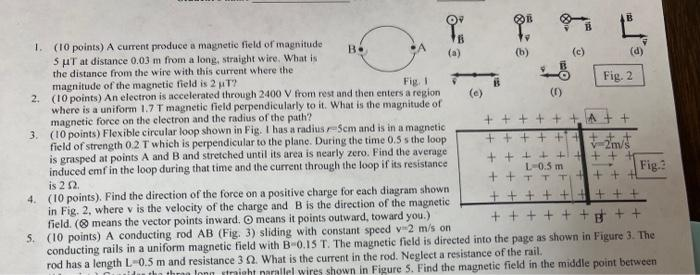# (Solved): answer5 1. (10 points) A current produce a magnetic field of magnitude 5T at distance 0.03m from ...1. (10 points) A current produce a magnetic field of magnitude at distance from a long, straight wire. What is the distance from the wire with this current where the magnitude of the magnetic field is ? 2. (10 points) An electron is accelerated through from rest and then enters a region where is a uniform 1.7 T magnetic field perpendicularly to it. What is the magnitude or magnetic force on the electron and the radius of the path? 3. (10 points) Flexible circular loop shown in . has a radius, and is in a magnetic field of strength which is perpendicular to the plane. During the time the loop is grasped at points A and B and stretched until its area is nearly zero. Find the average induced emf in the loop during that time and the current through the loop if its resistance is . 4. ( 10 points). Find the direction of the force on a positive charge for each diagram shown in Fig. 2, where is the velocity of the charge and is the direction of the magnetic field. ( means the vector points inward, means it points outward, toward you.) 5. (10 points) conducting rod (Fig. 3) sliding with constant speed on conducting rails in a uniform magnetic field with . The magnetic field is directed into the page as shown in Figure 3 . The rod has a length and resistance . What is the current in the rod. Neglect a resistance of the rail.

We have an Answer from Expert